(TL;DR – Create a measure which calculates the `SELECTEDVALUE` and use that rather than the table column to create the matrix)

We have recently been doing a lot of work with Power BI. In the process we came across a few things we thought it was worth sharing. The first of these is a quick tip on how to remove summarisation from columns in a matrix.

So, say we had data that looks like this: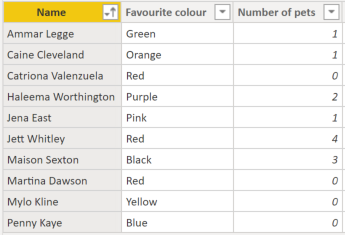If we then put that data into a matrix we get: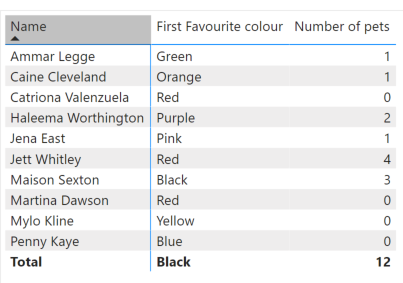Clearly the favourite colour column should not be being summarised. The issue is that the way that the matrix is being generated is that each row is applying a filter context – e.g. `[Name] = 'Ammar Legge'`. The favourite colour column is then just taking the first colour it finds. This works for each of the normal rows because there will only ever be a single row from the original table returned by each filter context.However, for the total column the same calculation is being carried out without any filter on name, and we are therefore just returning the colour which is first in the alphabet.

My first thought was that setting this: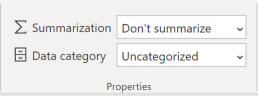On the favourite colour column would fix the problem. However, the matrix isn't using the default summarisation to calculate the values so this has no effect.Instead we need to create a measure: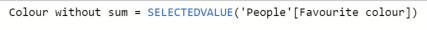And use that instead of the table column to create the matrix: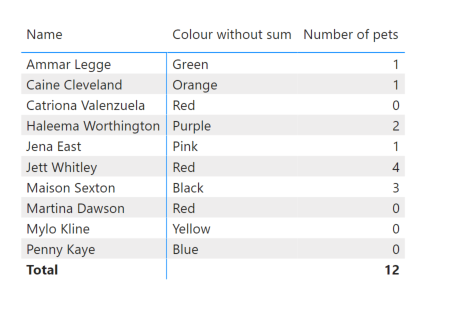This will then calculate the `SELECTEDVALUE` for each row, which will result in a `BLANK` when there isn't a single value for the column in the current filter context.

### Carmel Eve

#### Software Engineer I